Ch 2. Particle Force and Acceleration Multimedia Engineering Dynamics Rect.Coord. Normal/Tang. Coord. PolarCoord. Orbital Mechanics Computational Mechanics
 Chapter - Particle - 1. General Motion 2. Force & Accel. 3. Energy 4. Momentum - Rigid Body - 5. General Motion 6. Force & Accel. 7. Energy 8. Momentum 9. 3-D Motion 10. Vibrations Appendix Basic Math Units Basic Equations Sections Search eBooks Dynamics Fluids Math Mechanics Statics Thermodynamics Author(s): Kurt Gramoll ©Kurt GramollDYNAMICS - THEORY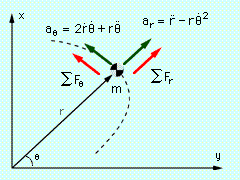Transverse Direction

In the previous chapter, the Curvilinear Motion: Polar Coordinates section developed expressions for acceleration in polar coordinates,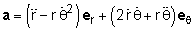where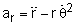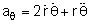Using this representation of acceleration, Newton's Second Law can be expressed in terms of polar coordinates, giving

ΣFrer + ΣFθeθ = m (arer + aθeθ)

The full relationship is: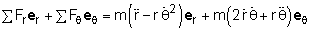Or, each of the two directions can be separated, giving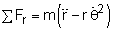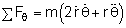Practice Homework and Test problems now available in the 'Eng Dynamics' mobile app
Includes over 400 problems with complete detailed solutions.
Available now at the Google Play Store and Apple App Store.